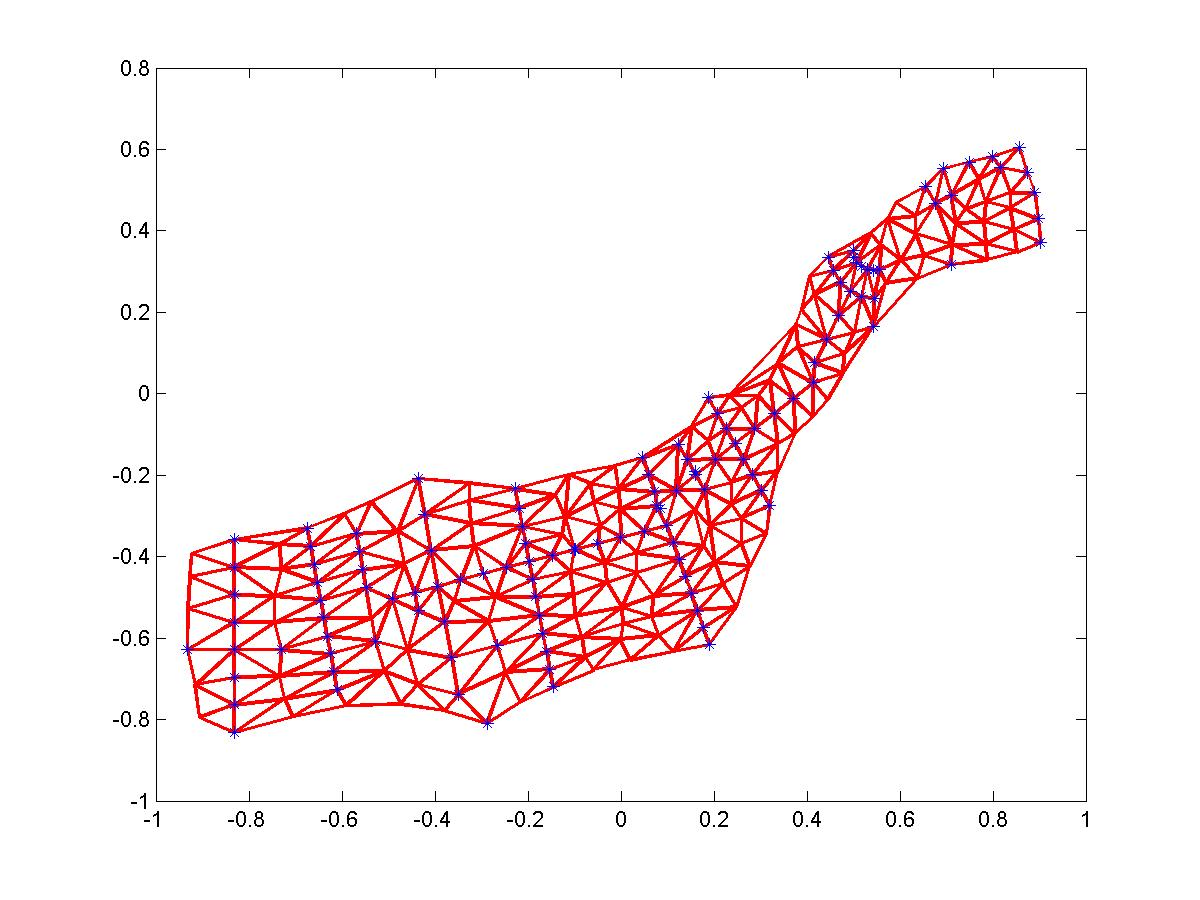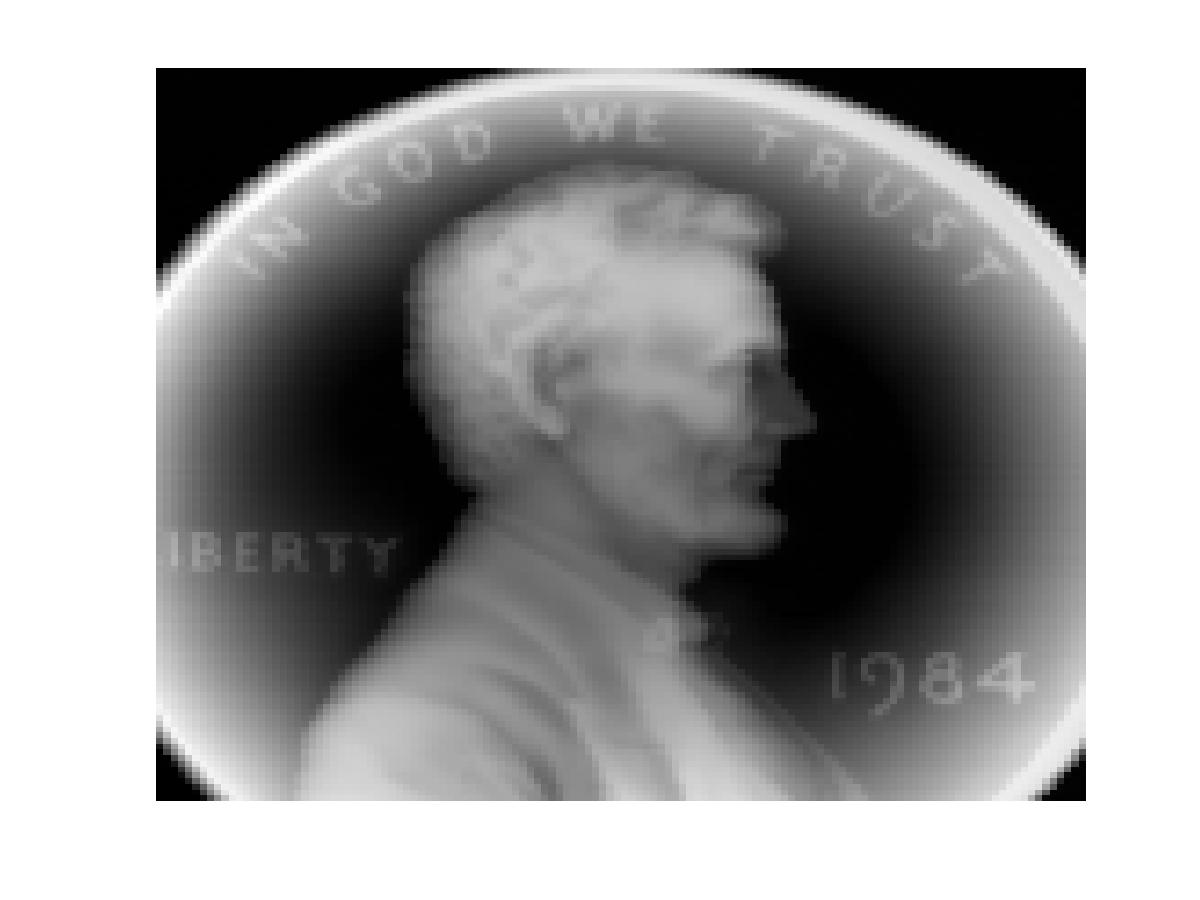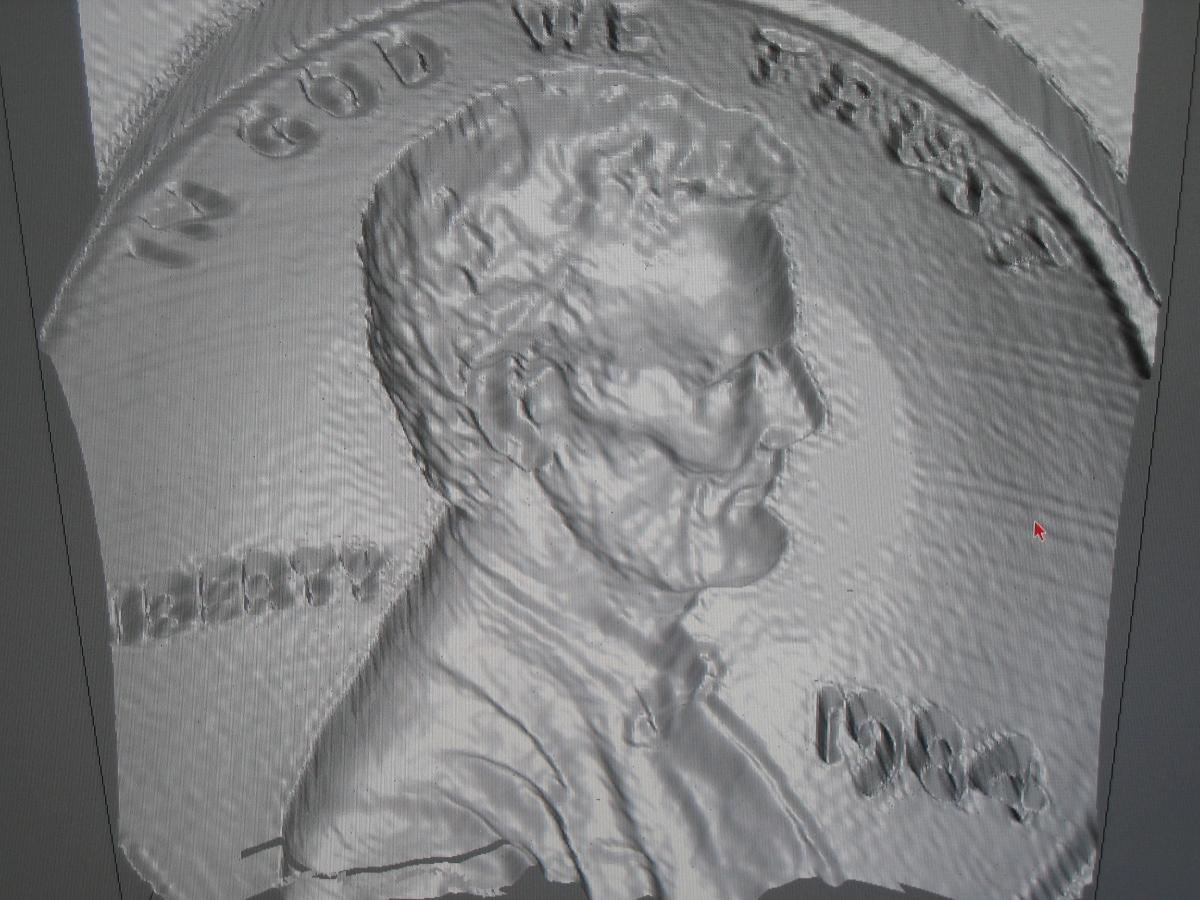# Scattered Data Interpolation and Fitting

Bivariate Splines are a good tool for interpolating and fitting any given values over scattered locations. That is, suppose we are given a set of data values (x_i, y_i, z_i), i=1, 2, ..., n. One is interested in finding a smooth surface S satisfying S(x_i,y_i)=z_i, i=1, ..., n.  Then bivariate splines can find such a surface easily. The following is a few examples.

Example 1:  I got a data set from a Marine Scientist Dr. Christof Meile at University of Georgia on the oxygen anomaly values over the polluted area by BP oil disaster in 2010. The data locations are shown in blue * in folloing figure with a triangulation. I use a nonnegative preserving interpolatory spline to find a smooth surface to interpolate the data values in mg/L as shown in the figure below.Example 2: I got a data set of car panel from Prof. Gerald Farin of Computer Science Dept. Arizona State Univ.. See the first image below. C1 cubic splines were used to find a surface which interpolates the data. The interpolatory spline gives the surface of a side panel of a car. One can see that the spline surface interpolates the given data as shown in the second surface (blue stars are given data points in 3D).Example 3: I got a set of scattered data which were sampled from a part of an airplane from Dr. Tom Grandine of Boeing Co.  See the data set below. Then I used spherical splines to find a C^1 smooth surface to interpolate the given data.(The detailed computation was done by my former Ph.D. student Victoria Baramidze). The interpolatory spline gives surface shown below. One can see that it fits the data very well.

Example 4: I got a penny image from MATLAB (load penny) and use C^1 qunitic spline to fit thepenny data (128x128) based on domain decomposition method (a joint work with L. L. Schumaker). I show two angles of the fitting surfaces below. This realizes a 3D visualization of 2D images.Example 5: I got a set of scattered data of geo-potential around Earth sampled by a satellite called CHAMP from Geoscientist C.K. Shum and his team, we used a spherical spline to fitting the data set. Let me first show the data locations around the Earth and a uniform triangulation of the Earth.The above is the spherical spline surface which can be seen very close to the given data values over the earth as in the third figure.